# Singular homology

(diff) ← Older revision | Latest revision (diff) | Newer revision → (diff)

The homology of a topological spacedefined using singular simplices in the same way as ordinary (simplicial) homology (and cohomology) of a polyhedron is defined using linear simplices. By a singular simplex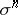one means a continuous mapping of an-dimensional standard simplexinto; the image ofis usually called the support ofand is denoted by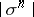. Singular chains are formal linear combinations of singular simplices with coefficients in an Abelian group. They form a groupwhich is isomorphic to the direct sum (over all) of the groups. Taken together, the chain groups form a singular chain complex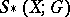with boundary homomorphism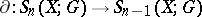given by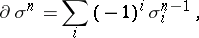where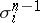is the composite withof the standard covering by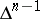of the-th face of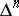. As usual, cycles and boundaries are those chains which belong to the kernel and image of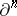, respectively. The-dimensional singular homology group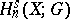is defined as the quotient group of the group of-dimensional cycles by the subgroup of boundaries.

If, then the groups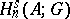are defined using the subcomplex ofconsisting of all chains with supports in, and groups of a pair,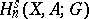, by using the corresponding quotient complex. There is an exact homology sequence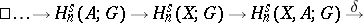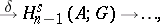which is a covariant functor on the category of pairsof topological spaces and their continuous mappings.

The homomorphismis defined as the boundary inof a cycle of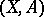representing the corresponding element of. Singular homology is homology with compact supports, in the sense that the groups associated withare equal to the direct limits of the homology groups of the compact sets.

Singular cohomology is defined in a dual way. The cochain complexis defined as the complex of homomorphisms intoof the integral singular chain complex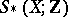. Less formally, cochains are functionsdefined on singular simplices and taking values in, and the co-boundary homomorphismis given by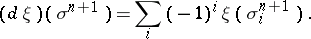The singular cohomology group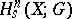is the quotient group of the group of-dimensional cocycles (the kernel of) by the subgroup of coboundaries (the image of). The cochain groups of a subspaceare defined by using the restriction of the cochain groups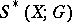to, while the cohomology groups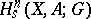of a pair are defined by using the cohomology of the subcomplex ofconsisting of all cochains which vanish on the singular simplices of. There is an exact sequencewhich is a contravariant functor of. The mappingis defined as the coboundary inof a cocycle ofrepresenting the corresponding element of.

Homology and cohomology groups with coefficients in an arbitrary groupcan be expressed in terms of integral homology and cohomology groups using universal coefficient formulas. In the case of cohomology, this formula is valid only whenis finitely generated.

In the category of polyhedra, the singular theory is equivalent to the simplicial (and also the cellular) theory. This fact is commonly used to establish the topological invariance of the latter. However, the importance of the singular homology groups is not limited to this. Being defined in a simple way, they are applicable to a fairly wide category of topological spaces and are homotopy invariants. The natural connections with homotopy theory make the singular theory indispensable to homotopical topology.

However, although singular homology groups are defined for all topological spaces without any restriction, their application is only justified under such restrictions as local contractibility or homological local connectedness. Singular chains, being by their nature "too" linearly connected, do not carry information about "continuous" cycles if the latter are not "sufficiently" linearly connected. There are other possible "anomalies" (for example, the homology groups of a compact subspace of Euclidean space can differ from zero in arbitrarily high dimensions, the homology and cohomology groups of a paircan be mapped non-isomorphically under the natural mapping fromonto the quotient space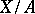corresponding to a closed subset, etc.). Therefore, in general categories of topological spaces one also uses the corresponding Aleksandrov–Čech cohomology and its associated homology (cf. Aleksandrov–Čech homology and cohomology). These theories are free from the above deficiencies and coincide with the singular one, at least in every case when its application does not raise problems.

How to Cite This Entry:
Singular homology. Encyclopedia of Mathematics. URL: http://encyclopediaofmath.org/index.php?title=Singular_homology&oldid=19148
This article was adapted from an original article by E.G. Sklyarenko (originator), which appeared in Encyclopedia of Mathematics - ISBN 1402006098. See original article